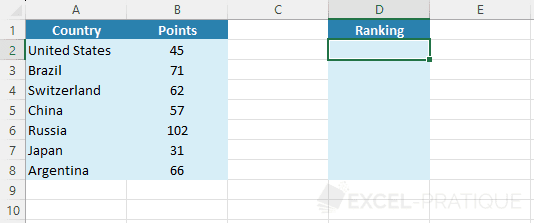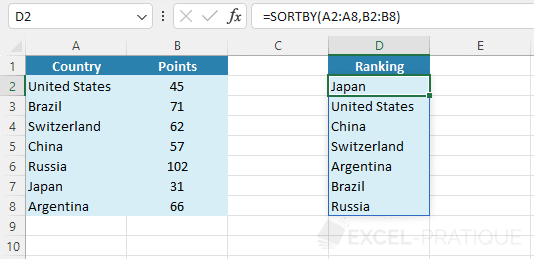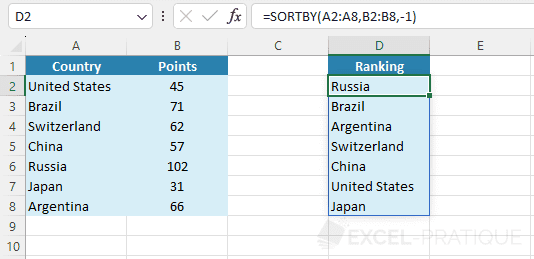# Excel Function: SORTBY

The Excel function SORTBY sorts the contents of a range of cells or a table based on a range of cells or another table.

This function allows for more advanced sorts than the SORT function (which only allows for sorting a table based on data contained within the same table).

Usage:

`=SORTBY(array, sort_array)`

or

`=SORTBY(array, sort_array_1, order_1, sort_array_2, order_2, ...)`

## Example of Use

In this example, the goal is to rank countries based on their number of points:Select the SORTBY function and then enter the cell range to return (that of the countries) as well as the cell range to use for the sort (that of the points):

``=SORTBY(A2:A8,B2:B8)``By default the data are sorted in ascending order, to perform a descending sort add -1 as the third argument:

``=SORTBY(A2:A8,B2:B8,-1)``